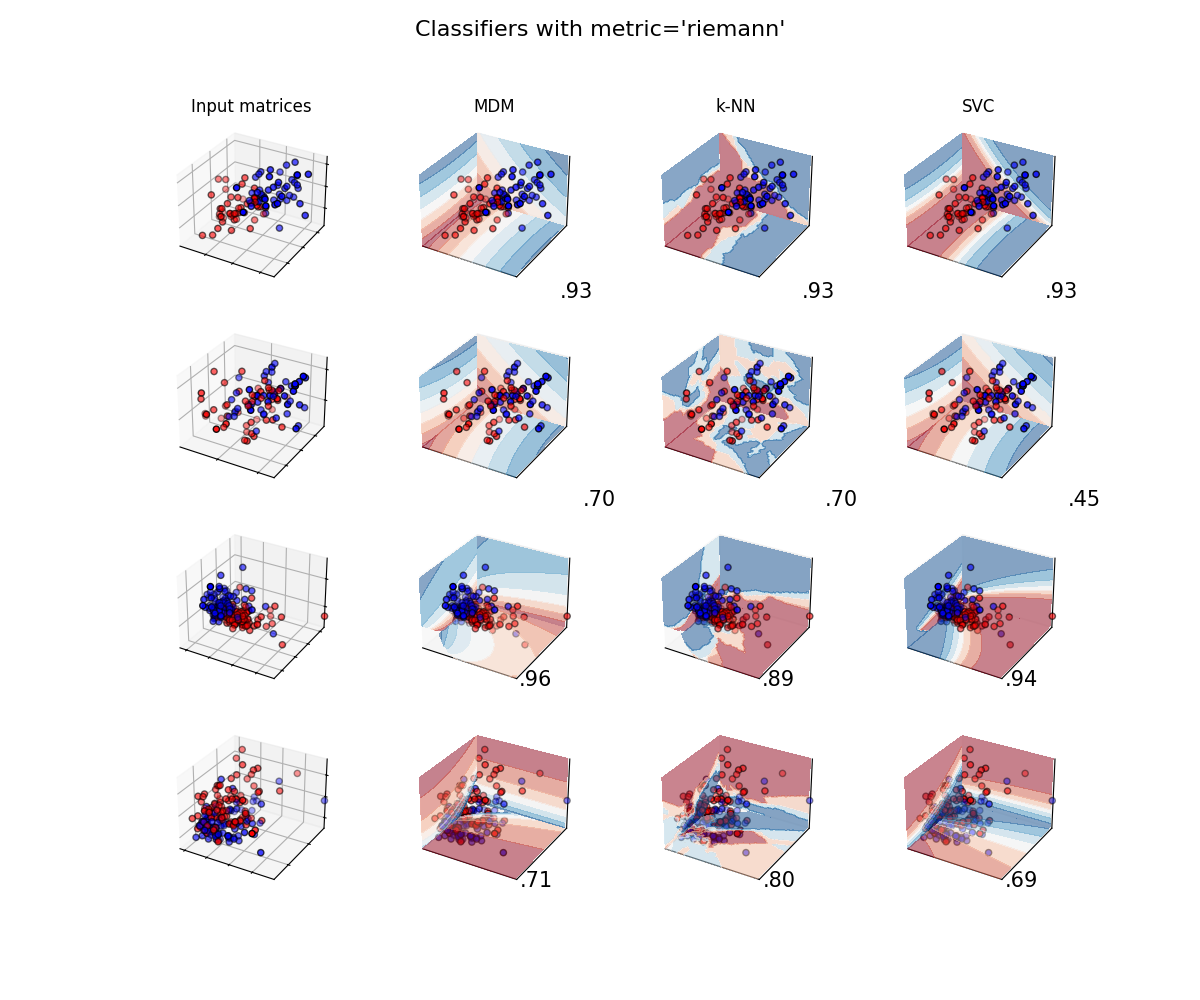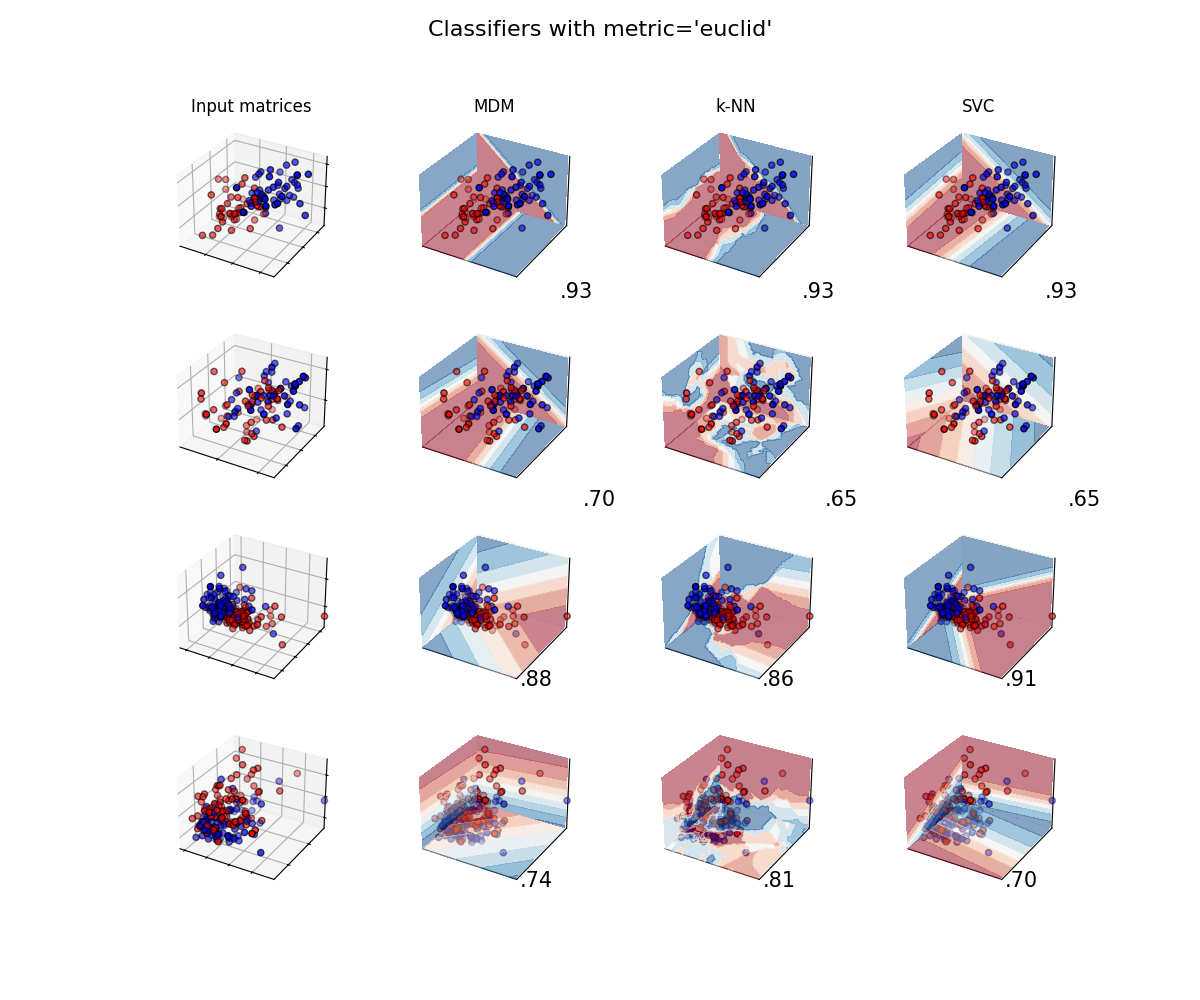# Classifier comparison¶

A comparison of several classifiers on low-dimensional synthetic datasets, adapted to SPD matrices from . The point of this example is to illustrate the nature of decision boundaries of different classifiers, used with different metrics . This should be taken with a grain of salt, as the intuition conveyed by these examples does not necessarily carry over to real datasets.

The 3D plots show training matrices in solid colors and testing matrices semi-transparent. The lower right shows the classification accuracy on the test set.

```# Authors: Quentin Barthélemy
#

from functools import partial
from time import time

import numpy as np
import matplotlib.pyplot as plt
from matplotlib.colors import ListedColormap
from sklearn.model_selection import train_test_split

from pyriemann.datasets import make_covariances, make_gaussian_blobs
from pyriemann.classification import (
MDM,
KNearestNeighbor,
SVC,
MeanField,
)
```
```@partial(np.vectorize, excluded=['clf'])
def get_proba(cov_00, cov_01, cov_11, clf):
cov = np.array([[cov_00, cov_01], [cov_01, cov_11]])
with np.testing.suppress_warnings() as sup:
sup.filter(RuntimeWarning)
return clf.predict_proba(cov[np.newaxis, ...])[0, 1]

def plot_classifiers(metric):
figure = plt.figure(figsize=(12, 10))
figure.suptitle(f"Compare classifiers with metric='{metric}'", fontsize=16)
i = 1

# iterate over datasets
for ds_cnt, (X, y) in enumerate(datasets):
# split dataset into training and test part
X_train, X_test, y_train, y_test = train_test_split(
X, y, test_size=0.4, random_state=42
)

x_min, x_max = X[:, 0, 0].min(), X[:, 0, 0].max()
y_min, y_max = X[:, 0, 1].min(), X[:, 0, 1].max()
z_min, z_max = X[:, 1, 1].min(), X[:, 1, 1].max()

# just plot the dataset first
ax = plt.subplot(n_datasets, n_classifs + 1, i, projection='3d')
if ds_cnt == 0:
ax.set_title("Input data")
# Plot the training points
ax.scatter(
X_train[:, 0, 0],
X_train[:, 0, 1],
X_train[:, 1, 1],
c=y_train,
cmap=cm_bright,
edgecolors="k"
)
# Plot the testing points
ax.scatter(
X_test[:, 0, 0],
X_test[:, 0, 1],
X_test[:, 1, 1],
c=y_test,
cmap=cm_bright,
alpha=0.6,
edgecolors="k"
)
ax.set_xlim(x_min, x_max)
ax.set_ylim(y_min, y_max)
ax.set_zlim(z_min, z_max)
ax.set_xticklabels(())
ax.set_yticklabels(())
ax.set_zticklabels(())
i += 1

rx = np.arange(x_min, x_max, (x_max - x_min) / 50)
ry = np.arange(y_min, y_max, (y_max - y_min) / 50)
rz = np.arange(z_min, z_max, (z_max - z_min) / 50)

print(f"Dataset n°{ds_cnt+1}")

# iterate over classifiers
for name, clf in zip(names, classifiers):
ax = plt.subplot(n_datasets, n_classifs + 1, i, projection='3d')

clf.set_params(**{'metric': metric})
t0 = time()
clf.fit(X_train, y_train)
t1 = time() - t0

t0 = time()
score = clf.score(X_test, y_test)
t2 = time() - t0

print(
f" {name}:\n  training time={t1:.5f}\n  test time    ={t2:.5f}"
)

# Plot the decision boundaries for horizontal 2D planes going
# through the mean value of the third coordinates
xx, yy = np.meshgrid(rx, ry)
zz = get_proba(xx, yy, X[:, 1, 1].mean()*np.ones_like(xx), clf=clf)
ax.contourf(xx, yy, zz, zdir='z', offset=z_min, cmap=cm, alpha=0.5)

xx, zz = np.meshgrid(rx, rz)
yy = get_proba(xx, X[:, 0, 1].mean()*np.ones_like(xx), zz, clf=clf)
ax.contourf(xx, yy, zz, zdir='y', offset=y_max, cmap=cm, alpha=0.5)

yy, zz = np.meshgrid(ry, rz)
xx = get_proba(X[:, 0, 0].mean()*np.ones_like(yy), yy, zz, clf=clf)
ax.contourf(xx, yy, zz, zdir='x', offset=x_min, cmap=cm, alpha=0.5)

# Plot the training points
ax.scatter(
X_train[:, 0, 0],
X_train[:, 0, 1],
X_train[:, 1, 1],
c=y_train,
cmap=cm_bright,
edgecolors="k"
)
# Plot the testing points
ax.scatter(
X_test[:, 0, 0],
X_test[:, 0, 1],
X_test[:, 1, 1],
c=y_test,
cmap=cm_bright,
edgecolors="k",
alpha=0.6
)

if ds_cnt == 0:
ax.set_title(name)
ax.text(
1.3 * x_max,
y_min,
z_min,
("%.2f" % score).lstrip("0"),
size=15,
horizontalalignment="right",
verticalalignment="bottom"
)
ax.set_xlim(x_min, x_max)
ax.set_ylim(y_min, y_max)
ax.set_zlim(z_min, z_max)
ax.set_xticks(())
ax.set_yticks(())
ax.set_zticks(())

i += 1

plt.show()
```

## Classifiers and Datasets¶

```names = [
"MDM",
"k-NN",
"SVC",
"MeanField",
]
classifiers = [
MDM(),
KNearestNeighbor(n_neighbors=3),
SVC(probability=True),
MeanField(power_list=[-1, 0, 1]),
]
n_classifs = len(classifiers)

rs = np.random.RandomState(2022)
n_matrices, n_channels = 50, 2
y = np.concatenate([np.zeros(n_matrices), np.ones(n_matrices)])

datasets = [
(
np.concatenate([
make_covariances(
n_matrices, n_channels, rs, evals_mean=10, evals_std=1
),
make_covariances(
n_matrices, n_channels, rs, evals_mean=15, evals_std=1
)
]),
y
),
(
np.concatenate([
make_covariances(
n_matrices, n_channels, rs, evals_mean=10, evals_std=2
),
make_covariances(
n_matrices, n_channels, rs, evals_mean=12, evals_std=2
)
]),
y
),
make_gaussian_blobs(
2*n_matrices, n_channels, random_state=rs, class_sep=1., class_disp=.2,
n_jobs=4
),
make_gaussian_blobs(
2*n_matrices, n_channels, random_state=rs, class_sep=.5, class_disp=.5,
n_jobs=4
)
]
n_datasets = len(datasets)

cm = plt.cm.RdBu
cm_bright = ListedColormap(["#FF0000", "#0000FF"])
```

## Classifiers with Riemannian metric¶

```plot_classifiers("riemann")
``````Dataset n°1
MDM:
training time=0.00224
test time    =0.00791
k-NN:
training time=0.00007
test time    =0.15394
SVC:
training time=0.00459
test time    =0.00223
MeanField:
training time=0.00279
test time    =0.02555
Dataset n°2
MDM:
training time=0.00229
test time    =0.00794
k-NN:
training time=0.00007
test time    =0.15469
SVC:
training time=0.00525
test time    =0.00227
MeanField:
training time=0.00285
test time    =0.02358
Dataset n°3
MDM:
training time=0.00577
test time    =0.01421
k-NN:
training time=0.00005
test time    =0.45601
SVC:
training time=0.00708
test time    =0.00247
MeanField:
training time=0.00652
test time    =0.04557
Dataset n°4
MDM:
training time=0.00648
test time    =0.01437
k-NN:
training time=0.00007
test time    =0.45662
SVC:
training time=0.00904
test time    =0.00252
MeanField:
training time=0.00742
test time    =0.04568
```

## Classifiers with Log-Euclidean metric¶

```plot_classifiers("logeuclid")
``````Dataset n°1
MDM:
training time=0.00095
test time    =0.01147
k-NN:
training time=0.00007
test time    =0.21000
SVC:
training time=0.00299
test time    =0.00206
MeanField:
training time=0.00290
test time    =0.03548
Dataset n°2
MDM:
training time=0.00093
test time    =0.01153
k-NN:
training time=0.00007
test time    =0.20819
SVC:
training time=0.00327
test time    =0.00203
MeanField:
training time=0.00291
test time    =0.03552
Dataset n°3
MDM:
training time=0.00110
test time    =0.02145
k-NN:
training time=0.00007
test time    =0.66308
SVC:
training time=0.00358
test time    =0.00223
MeanField:
training time=0.00658
test time    =0.06925
Dataset n°4
MDM:
training time=0.00128
test time    =0.02205
k-NN:
training time=0.00008
test time    =0.66839
SVC:
training time=0.00450
test time    =0.00232
MeanField:
training time=0.00731
test time    =0.06932
```

## Classifiers with Euclidean metric¶

```plot_classifiers("euclid")
``````Dataset n°1
MDM:
training time=0.00044
test time    =0.00279
k-NN:
training time=0.00007
test time    =0.04682
SVC:
training time=0.00232
test time    =0.00146
MeanField:
training time=0.00291
test time    =0.00807
Dataset n°2
MDM:
training time=0.00044
test time    =0.00280
k-NN:
training time=0.00007
test time    =0.05093
SVC:
training time=0.00379
test time    =0.00164
MeanField:
training time=0.00289
test time    =0.00806
Dataset n°3
MDM:
training time=0.00047
test time    =0.00445
k-NN:
training time=0.00006
test time    =0.14049
SVC:
training time=0.00273
test time    =0.00158
MeanField:
training time=0.00635
test time    =0.01489
Dataset n°4
MDM:
training time=0.00042
test time    =0.00439
k-NN:
training time=0.00009
test time    =0.13930
SVC:
training time=0.00367
test time    =0.00162
MeanField:
training time=0.00740
test time    =0.01470
```